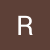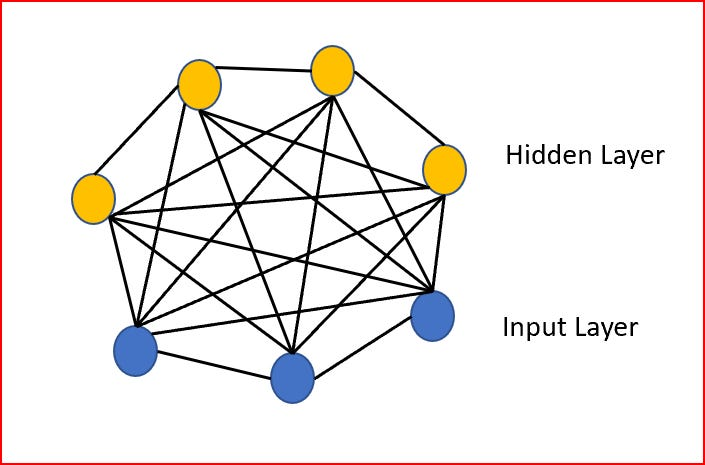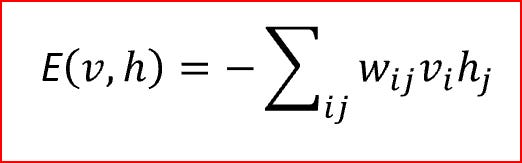# Deep Learning — Restricted Boltzmann Machine

Source: Deep Learning on Medium

In this post we will discuss Boltzmann Machine, Restricted Boltzmann machine(RBM). Need for RBM, RBM architecture, usage of RBM and KL divergence. We will explain how recommender systems work using RBM with an example

#### What is Boltzmann Machine?

• Boltzmann machine is a network of symmetrically connected nodes
• Nodes makes stochastic decision, to be turned on or off.
• Boltzmann machine is an unsupervised machine learning algorithm. It helps discover latent features present in the dataset. Dataset is composed of binary vectors.
• Connection between nodes are undirected. Each node in Boltzmann machine is connected to every other node.
• We have input layer and hidden layer but no output layer. This because each node is treated as same. All nodes are part of the system. Each node generate states of the system and hence it is a generative model.
• We input the data into Boltzmann machine. Model helps learn different connection between nodes and weights of the parameters.
• Boltzmann machine is an energy based model
• Boltzmann machine can be compared to a greenhouse. In greenhouse we need to different parameters monitor humidity, temperature, air flow, light
• Like Boltzmann machine, greenhouse is a system. For green house we learn relationship between humidity, temperature, light and airflow. Understanding of the relationship between different parameters like humidity, airflow, soil condition etc. hep us understand the impact on the greenhouse yield.Boltzmann machine

#### Why Restricted Boltzmann machine?

In Boltzmann machine, each node is connected to every other node.. Connection between all nodes are undirected. Boltzmann machine has not been proven useful for practical machine learning problems .

Boltzmann machine can be made efficient by placing certain restrictions. Restrictions like no intralayer connection in both visible layer and hidden layer.

#### What is Restricted Boltzmann Machine?

• RBM are neural network that belong to energy based model
• It is probabilistic, unsupervised, generative deep machine learning algorithm.
• RBM’s objective is to find the joint probability distribution that maximizes the log likelihood function.
• RBM is undirected and have only two layers, Input layer and hidden layer
• All visible nodes are connected to all the hidden nodes. RBM it has two layers, visible layer or input layer and hidden layer so it is also called as symmetrical bipartite graph.
• No intra layer connection exists between the visible nodes. There is also no intra layer connection between the hidden nodes. There are connections only between input and hidden nodes.
• Original Boltzmann machine had connections between all the nodes. Since RBM restricts the intra layer connection, it is called as Restricted Boltzmann Machine
• Like Boltzmann machine, RBM nodes also make stochastic decision to decide either to be turned on or off
• RBM is energy based model with joint probabilities like Boltzmann machinesEnergy function for Restricted Boltzmann Machine. V is vector for visible or input node, h is vector for hidden node and W is the weights between the input and hidden nodes

#### Architecture of Restricted Boltzmann Machine

We pass the input data from each of the visible node to the hidden layer.

We multiply the input data by the weight assigned to the hidden layer, add the bias term and applying an activation function like sigmoid or softmax activation function.

Forward propagation gives us probability of output for a given weight w ,this gives P(a|x) for weights w.

During back propagation we reconstruct the input. During reconstruction RBM estimates the probability of input x given activation a, this gives us P(x|a) for weight w.

We can derive the joint probability of input x and activation a, P(x,a)

Reconstruction is about the probability distribution of the original input.

We compare the difference between input and reconstruction using KL divergence.

#### Kullback Leibler(KL) Divergence

• KL divergence measures the difference between two probability distribution over the same data x.
• It is a non symmetrical measure between the two probabilities p(x) and q(x). It is a measure of information loss when we use a distribution q(x) to approximate a distribution p(x)
• KL divergence measures the distance between two distributions. It is not the distance measure as KL divergence is not a metric measure and does not satisfy the triangle inequality

KL divergence can be calculated using the below formula

Here we have two probability distribution p(x) and q(x) for data x. Both p(x) and q(x) sum upto to 1 and p(x) >0 and q(x)>0.

p(x) is the true distribution of data and q(x) is the distribution based on our model, in our case RBM.

If the model distribution is same as the true distribution, p(x)=q(x)then KL divergence =0

#### Usage of RBM

RBM’s are used for

• Dimensionality reduction
• Collaborative filtering for recommender systems
• Feature learning
• Topic modelling
• Helps improve efficiency of Supervised learning

Let’s under intuitively how is RBM used.

#### How does RBM learn hidden features?

Step 1:Take input vector to the visible node

Step 2:Update the weights of all hidden nodes in parallel

Step 3: Reconstruct the input vector

Step 4: Compare the input to the reconstructed input based on KL divergence.

Step 5: Based on error, adjust the weights of the hidden layer accordingly

Step 6: Reconstruct the input vector again and keep repeating for all the input data and for multiple epochs. This process is repeated till we get the minimal reconstruction error.

#### How RBM can be used to Recommend Products?

In our example, we have 5 products and 5 customer. In real life we will have large set of products and millions of customers buying those products. A value of 1 represents that the Product was bought by the customer. A value of 0 represents that the product was not bought by the customer.

We know that RBM is generative model and generate different states. In doing so it identifies the hidden features for the input dataset.

Highlighted data in red shows that some relationship between Product 1, Product 3 and Product 4. Different customers have bought these products together.

RBM assigns a node to take care of the feature that would explain the relationship between Product1, Product 3 and Product 4.

Based on the the input dataset RBM identifies three important features for our input data.

RBM identifies the underlying features based on what products were bought by the customer. Customer buy Product based on certain usage.

For our understanding, let’s name these three features as shown below.

Once the model is trained we have identified the weights for the connections between the input node and the hidden nodes.

Let’s take a customer data and see how recommender system will make recommendations.

Our Customer is buying Baking Soda. Based on the features learned during training, we see that hidden nodes for baking and grocery will have higher weights and they get lighted. Hidden node for cell phone and accessories will have a lower weight and does not get lighted.

During back propagation, RBM will try to reconstruct the input. During recommendation, weights are no longer adjusted. Weights derived from training are used while recommending products.

For our test customer, we see that the best item to recommend from our data is sugar. Sugar lights up both baking item hidden node and grocery hidden node.

Hope this basic example help understand RBM and how RBMs are used for recommender systems

#### References

https://www.cs.toronto.edu/~hinton/csc321/readings/boltz321.pdf

https://www.cs.toronto.edu/~rsalakhu/papers/rbmcf.pdf